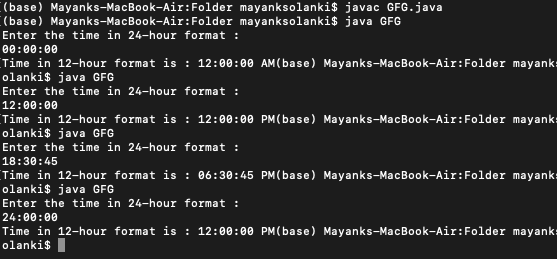Related Articles
Java Program to Format Time in AM-PM format
• Last Updated : 02 Dec, 2020

Date Time Class Format is used to display date and time and manipulate date and time in java and in addition to this it is also used for formatting date and time class in java across time zone associated data. So in order to import this class from a package called ‘java.utils’. After importing this class one can create an object of the Date class in order to print the current date and time. Now in order to print the default date and time simply call the print command using toString() method to get the current date and time.

Milliseconds if computed through inbuilt data class function call from any time in the code base reference is set as 1 Jan 1970. In order to print no of milliseconds till now simply use getTime() instead of to String() to get no of milliseconds till now. Suppose if the user wants a particular date, time, and month from the current time. This can be done by the Date and SimpleDateFormat classes of the java.

Note: Epoch time 1 Jan 1970

Approaches:

• Using SimpleDateFormat
• Using splitting the string

Approach 1: SimpleDateFormat

SimpleDateFormat class is a class in Java that provides several methods to parse and format the date and time. This class inherits java.text.DateFormat class. The format() method of DateFormat class in Java is used to format a given date into Date/Time string. Basically, the method is used to convert this date and time into a particular format i.e., “mm/dd/yyyy”.  format() method used to change the format of the parameters given to it in a particular manner.

Syntax:

`public final String format(Date date)`

Parameters: The method takes one parameter date of Date object type and refers to the date whose string output is to be produced.

Return Value: The method returns Date or time in string format of “mm/dd/yyyy”

Implementation: In this java example the time entered by the user is converted from 24 to 12hour formats with AM/PM marker.

## Java

 `// Java program to convert 24 hour ``// time format to 12 hour format`` ` `// Importing specific date class libraries``import` `java.util.Date;``import` `java.text.SimpleDateFormat;`` ` `public` `class` `GFG {``   ` `  ``// Main driver method``    ``public` `static` `void` `main(String[] args)``    ``{``        ``// Getting the current current time``        ``Date date = ``new` `Date();`` ` `       ` `        ``System.out.println(``"Current Time is : "` `+ date);`` ` `        ``// set format in 12 hours``        ``SimpleDateFormat formatTime = ``new` `SimpleDateFormat(``"hh.mm aa"``);``        ``// hh = hours in 12hr format``        ``// mm = minutes``        ``// aa = am/pm`` ` `        ``String time = formatTime.format(``            ``date); ``// changing the format of 'date'`` ` `        ``// display time as per format``        ``System.out.println(``            ``"Current Time in AM/PM Format is : "` `+ time);``    ``}``}`
Output
```Current Time is : Mon Oct 26 08:34:53 UTC 2020
Current Time in AM/PM Format is : 08.34 AM```

Approach 2: Without using any Special class of java

Here only inbuilt methods are used as listed below in tabular format and the role of them in conversion. Time is concerted with AM/PM markers in 12 formt standard from 24 hour format without even importing Date class.

Implementation:

## Java

 `// Java program to convert 24 hour ``// time format to 12 hour format`` ` `// Importing generic java libraries``import` `java.util.*;`` ` `class` `GFG {`` ` `    ``// Function converting entered values to String type``    ``static` `void` `convertTime(String time)``    ``{``        ``String format;`` ` `        ``// Parsing hours, minutes and seconds in array``        ``String[] arr = time.split(``":"``);`` ` `        ``// Converting hours into integer``        ``int` `hh = Integer.parseInt(arr[``0``]);`` ` `        ``if` `(hh > ``12``) {``            ``hh = hh - ``12``;``            ``format = ``"PM"``;``        ``}``        ``else` `if` `(hh == ``00``) {``            ``hh = ``12``;``            ``format = ``"AM"``;``        ``}``        ``else` `if` `(hh == ``12``) {``            ``hh = ``12``;``            ``format = ``"PM"``;``        ``}``        ``else` `{``            ``format = ``"AM"``;``        ``}`` ` `        ``// Converting hh to String and``        ``// padding it with 0 on left side``        ``String hour = String.format(``"%02d"``, hh);``        ``String minute = arr[``1``];``        ``String second = arr[``2``];`` ` `        ``// Printing formatted time``        ``System.out.print(``"Time in 12-hour format is : "``);``        ``System.out.print(hour + ``":"` `+ minute + ``":"` `+ second``                         ``+ ``" "` `+ format);``    ``}`` ` `    ``// Main driver code``    ``public` `static` `void` `main(String[] args)``    ``{``        ``// Taking input from the user via Scanner class``        ``Scanner sc = ``new` `Scanner(System.in);`` ` `        ``// Asking from user to enter``        ``// time in 24 format``        ``System.out.println(``            ``"Enter the time in 24-hour format : "``);`` ` `        ``/* User: Remember to enter time in below format``                 ``Enter in this format - '14:02:45' */``        ``String time = sc.nextLine();`` ` `        ``// Passing time as entered above as parameter``        ``// wher efunrion isn``        ``convertTime(time);``    ``}``}`

Output:Attention reader! Don’t stop learning now. Get hold of all the important Java Foundation and Collections concepts with the Fundamentals of Java and Java Collections Course at a student-friendly price and become industry ready. To complete your preparation from learning a language to DS Algo and many more,  please refer Complete Interview Preparation Course.

My Personal Notes arrow_drop_up